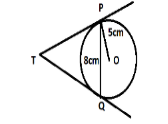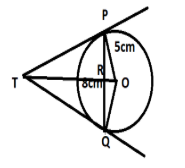QUESTION

# PQ is a chord of length 8 cm of a circle of radius 5 cm and centre O. The tangents at P and Q intersect at a point T. Find the length TP.Hint: Draw a line TR which intersects PQ at R. Also, OT is perpendicular to PQ. Therefore, we can write PR = QR. Apply Pythagoras theorem and then solve the question.

Complete step-by-step solution -
Given in the question, chord PQ = 8cm.
Radius of the circle = 5cm.
TP and TQ are the tangents to the circle through P and Q.
To find: The length of TP.
Now, using the hint, draw a line TR which intersect PQ at R. SO, the figure will be-Now let TR = y.
Since, OT is a perpendicular bisector of PQ.
We can say, PR = QR = 4cm.
Using the right triangle OTP and PTR, we have,
$T{P^2} = T{R^2} + P{R^2}$.
Also,
We use the concept that tangent at a point of a circle from any point which is outside to the circle is to be perpendicular to the radius ,Here OP is radius and TP is tangent at point P So we use pythagoras theorem for triangle TPO.
$O{T^2} = T{P^2} + O{P^2} \\ \Rightarrow O{T^2} = (T{R^2} + P{R^2}) + O{P^2} \to (1) \\$
Now, OT = OR + TR.
We know, OR = 3cm, TR = y (say).
Also, $O{R^2} = O{P^2} - P{R^2}$
Therefore, equation (1) will become –
$\Rightarrow {(y + 3)^2} = ({y^2} + 16 + 25) \\ \Rightarrow {y^2} + 9 + 6y = {y^2} + 16 + 25 \\ \Rightarrow 6y = 32 \\ \therefore y = \dfrac{{16}}{3} \\$
So, $y = TR = \dfrac{{16}}{3}$
We can write that, $T{P^2} = T{R^2} + P{R^2} \\ \Rightarrow T{P^2} = {\left( {\dfrac{{16}}{3}} \right)^2} + {4^2} = \dfrac{{256}}{9} + 16 = \dfrac{{400}}{9} \\ \Rightarrow TP = \dfrac{{20}}{3}cm \\$.
Hence, the length of TP is 20/3 cm.

Note: Whenever such types of questions appear, then write down the information and the values given in the question. Then, use the figure to find relation between various variables. As mentioned in the solution to find TP apply Pythagoras theorem in right angle triangle OTP and PTR, then find the values of the unknown lengths and then solve the question further to find TP.